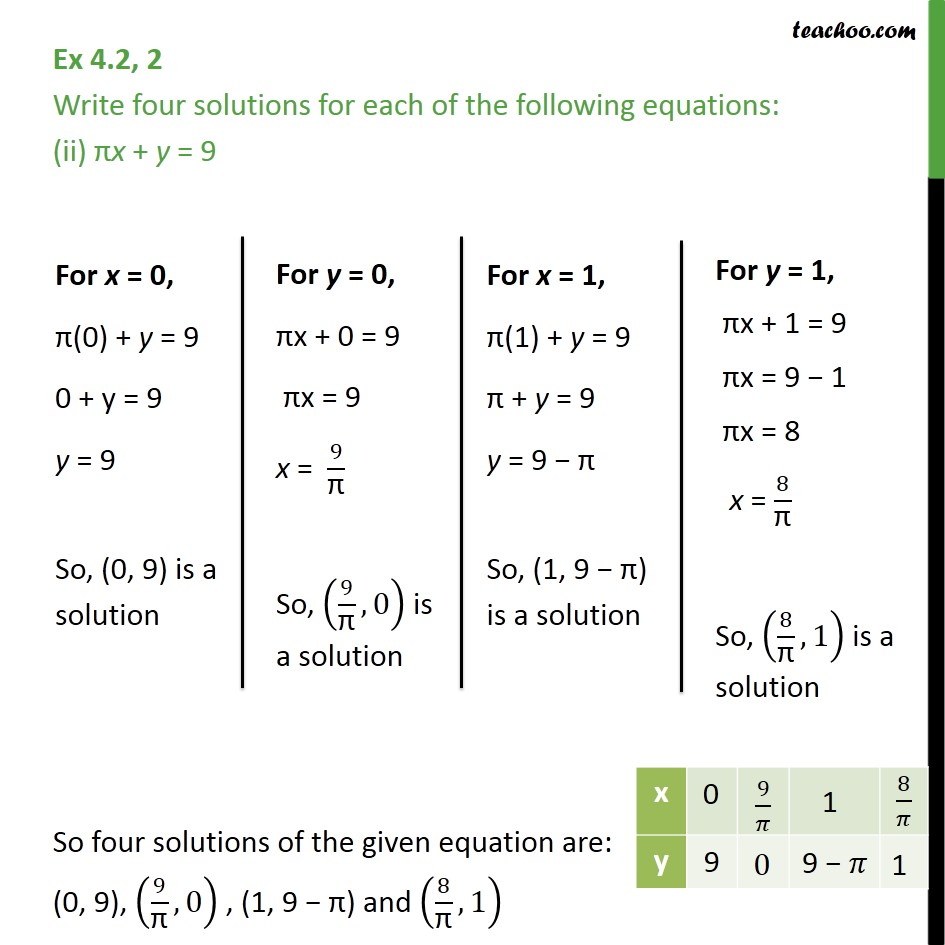Ex 4.2

Chapter 4 Class 9 Linear Equations in Two Variables
Serial order wiseLearn in your speed, with individual attention - Teachoo Maths 1-on-1 Class

### Transcript

Ex 4.2, 2 Write four solutions for each of the following equations: (ii) x + y = 9 So four solutions of the given equation are: (0, 9), (9/" " ,0) , (1, 9 ) and (8/" " ,1)